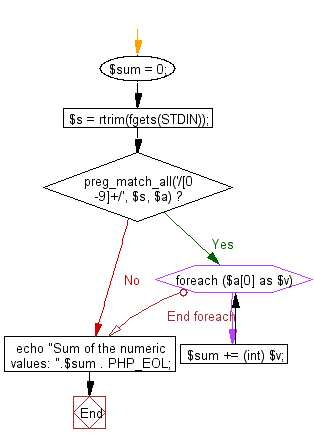﻿ PHP Exercise: Sum of all numerical values embedded in a sentence - w3resource

# PHP Exercises: Sum of all numerical values embedded in a sentence

## PHP: Exercise-69 with Solution

Write a PHP program to sum of all numerical values (positive integers) embedded in a sentence.
Sentences with positive integers are given over multiple lines. Each line is a character string containing one-byte alphanumeric characters, symbols, spaces, or an empty line. However the input is 80 characters or less per line and the sum is 10,000 or less.

Sample Solution: -

PHP Code:

``````<?php
\$sum = 0;
\$s = rtrim(fgets(STDIN));
if (preg_match_all('/[0-9]+/', \$s, \$a)) {
foreach (\$a as \$v) {
\$sum += (int) \$v;
}
}
echo "Sum of the numeric values: ".\$sum . PHP_EOL;
?>
```
```

Sample Input:
5 apple and 10 orange are rotten in the basket

Sample Output:

`Sum of the numeric values: 15`

Flowchart:PHP Code Editor:

Have another way to solve this solution? Contribute your code (and comments) through Disqus.

What is the difficulty level of this exercise?

Test your Programming skills with w3resource's quiz.

﻿

## PHP: Tips of the Day

Returns all elements in an array except for the first one

Example:

```<?php
function tips_tail(\$items)
{
return count(\$items) > 1 ? array_slice(\$items, 1) : \$items;
}

print_r(tips_tail([1, 5, 7]));
?>
```

Output:

```Array
(
 => 5
 => 7
)
```